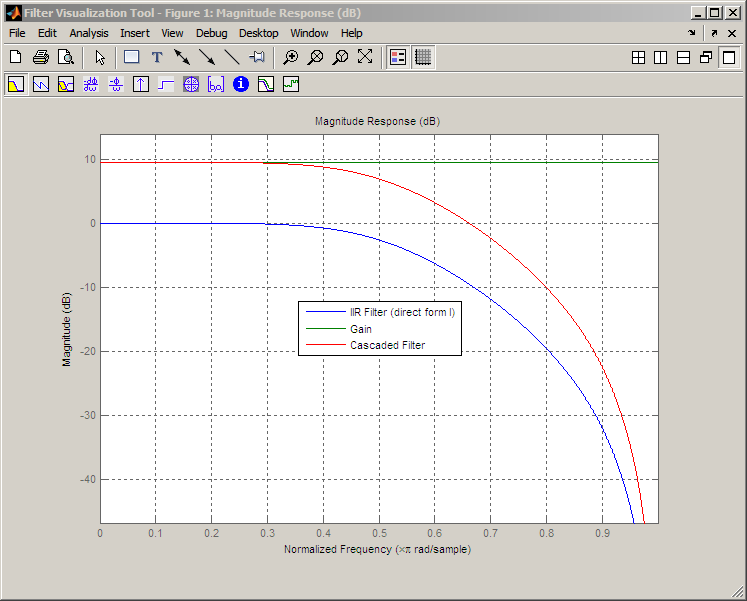# dfilt.scalar

Discrete-time, scalar filter

## Syntax

```dfilt.scalar(g) dfilt.scalar ```

## Description

`dfilt.scalar(g)` returns a discrete-time, scalar filter object with gain `g`, where `g` is a scalar.

Make this filter a fixed-point or single-precision filter by changing the value of the `Arithmetic` property for the filter `hd` as follows:

• To change to single-precision filtering, enter

`set(hd,'arithmetic','single');`
• To change to fixed-point filtering, enter

`set(hd,'arithmetic','fixed');`

For more information about the property `Arithmetic`, refer to Arithmetic.

`dfilt.scalar` returns a default, discrete-time scalar gain filter object `hd`, with gain `1`.

## Properties

In this table you see the properties associated with the scalar implementation of `dfilt` objects.

Note

The table lists all the properties that a filter can have. Many of the properties are dynamic, meaning they exist only in response to the settings of other properties. You might not see all of the listed properties all the time. To view all the properties for a filter at any time, use

`get(hd)`

where `hd` is a filter.

For further information about the properties of this filter or any `dfilt` object, refer to Fixed-Point Filter Properties.

Property Name

Brief Description

`Arithmetic`

Defines the arithmetic the filter uses. Gives you the options `double`, `single`, and `fixed`. In short, this property defines the operating mode for your filter.

`CastBeforeSum`

Specifies whether to cast numeric data to the appropriate accumulator format (as shown in the signal flow diagrams) before performing sum operations.

`CoeffAutoScale`

Specifies whether the filter automatically chooses the proper fraction length to represent filter coefficients without overflowing. Turning this off by setting the value to `false` enables you to change the `CoeffFracLength` property to specify the precision used.

`CoeffFracLength`

Set the fraction length the filter uses to interpret coefficients. `CoeffFracLength` is always available, but it is read-only until you set `CoeffAutoScale` to `false`.

`CoeffWordLength`

Specifies the word length to apply to filter coefficients.

`FilterStructure`

Describes the signal flow for the filter object, including all of the active elements that perform operations during filtering — gains, delays, sums, products, and input/output.

`Gain`

Returns the gain for the scalar filter. Scalar filters do not alter the input data except by adding gain.

`InputFracLength`

Specifies the fraction length the filter uses to interpret input data.

`InputWordLength`

Specifies the word length applied to interpret input data.

`OutputFracLength`

Determines how the filter interprets the filter output data. You can change the value of `OutputFracLength` when you set `OutputMode` to `SpecifyPrecision`.

`OutputMode`

Sets the mode the filter uses to scale the filtered data for output. You have the following choices:

• `AvoidOverflow` — directs the filter to set the output data word length and fraction length to avoid causing the data to overflow.

• `BestPrecision` — directs the filter to set the output data word length and fraction length to maximize the precision in the output data.

• `SpecifyPrecision` — lets you set the word and fraction lengths used by the output data from filtering.

`OutputWordLength`

Determines the word length used for the output data.

`OverflowMode`

Sets the mode used to respond to overflow conditions in fixed-point arithmetic. Choose from either `saturate` (limit the output to the largest positive or negative representable value) or `wrap` (set overflowing values to the nearest representable value using modular arithmetic). The choice you make affects only the accumulator and output arithmetic. Coefficient and input arithmetic always saturates. Finally, products never overflow — they maintain full precision.

`PersistentMemory`

Specifies whether to reset the filter states and memory before each filtering operation. Lets you decide whether your filter retains states from previous filtering runs. `False` is the default setting.

`RoundMode`

Sets the mode the filter uses to quantize numeric values when the values lie between representable values for the data format (word and fraction lengths).

• `ceil` - Round toward positive infinity.

• `convergent` - Round to the closest representable integer. Ties round to the nearest even stored integer. This is the least biased of the methods available in this software.

• `fix` - Round toward zero.

• `floor` - Round toward negative infinity.

• `nearest` - Round toward nearest. Ties round toward positive infinity.

• `round` - Round toward nearest. Ties round toward negative infinity for negative numbers, and toward positive infinity for positive numbers.

The choice you make affects only the accumulator and output arithmetic. Coefficient and input arithmetic always round. Finally, products never overflow — they maintain full precision.

`Signed`

Specifies whether the filter uses signed or unsigned fixed-point coefficients. Only coefficients reflect this property setting.

`States`

This property contains the filter states before, during, and after filter operations. States act as filter memory between filtering runs or sessions. The states use `fi` objects, with the associated properties from those objects. For details, refer to `filtstates` in Signal Processing Toolbox™ documentation or in the Help system.

## Examples

Create a direct-form I filter object `hd_filt` and a scalar object with a gain of 3 `hd_gain` and cascade them together.

```b = [0.3 0.6 0.3]; a = [1 0 0.2]; hd_filt = dfilt.df1(b,a); hd_gain = dfilt.scalar(3); hd_cascade=cascade(hd_gain,hd_filt); fvtool_handle = fvtool(hd_filt,hd_gain,hd_cascade); legend(fvtool_handle,'IIR Filter (direct form I)',... 'Gain','Cascaded Filter');```To view the stages of the cascaded filter, use

```hd.Stage(1) ```

and

```hd.Stage(2) ```